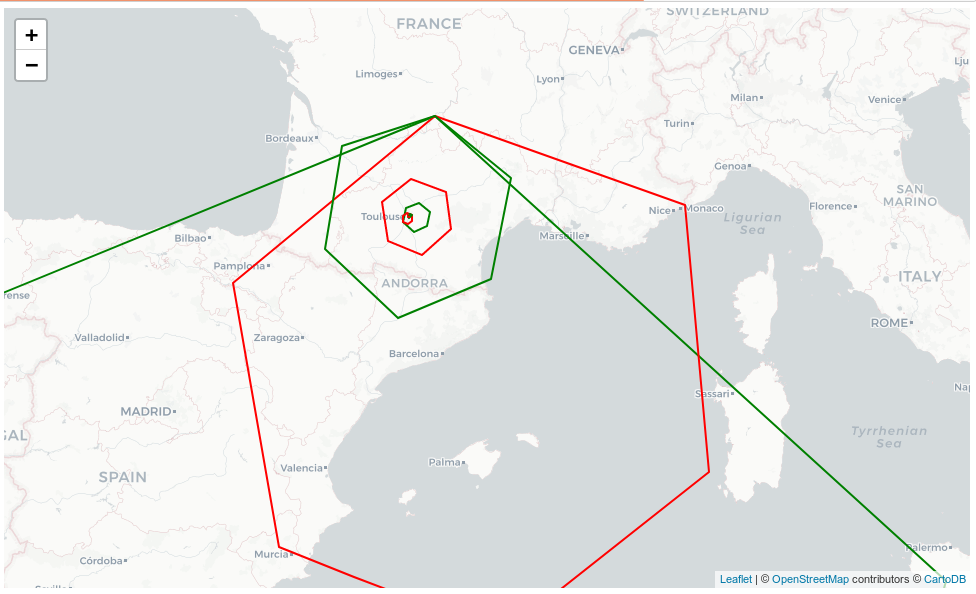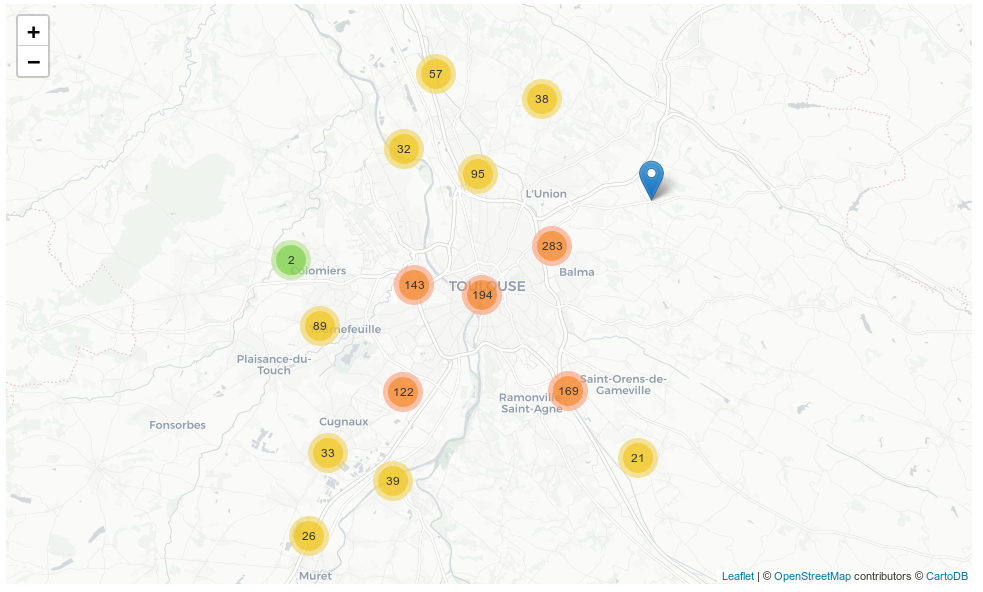## Uber H3 API examples on Urban Analytics in the city of Toulouse (France)¶

### Author: Camelia Ciolac¶

[email protected]

"The H3 geospatial indexing system is a multi-precision hexagonal tiling of the sphere indexed with hierarchical linear indexes." (https://github.com/uber/h3/blob/master/docs/overview/mainpage.md)

We use the following methods from H3 Python API:

• h3.edge_length
• h3.hex_area
• h3.geo_to_h3
• h3.h3_to_geo_boundary
• h3.h3_get_resolution
• h3.k_ring_distances
• h3.h3_distance
• h3.polyfill
• h3.compact
• h3.hex_ring

For data visualization we use:

• Folium
• deck.gl (Uber's large-scale WebGL-powered data visualization framework)

### Setup steps¶

Virtual environment was set up as follows:

virtualenv -p python3 ./projenv_demo_h3

source projenv_demo_h3/bin/activate

pip3 install ipython==7.2.0 jupyter==1.0.0

jupyter notebook
In :
%%sh
pip3 freeze | grep -E 'ipython|jupyter'

ipython==7.2.0
ipython-genutils==0.2.0
jupyter==1.0.0
jupyter-client==5.2.3
jupyter-console==6.0.0
jupyter-core==4.4.0


Now, we list this demo's dependencies in a file called requirements_demo.txt

In :
%%sh
cat <<EOF > requirements_demo.txt
h3==3.1.0
pandas>=0.23
geojson==2.4.1
folium==0.7.0
seaborn==0.9.0
area==1.1.1
statsmodels==0.9.0
EOF


Use pip to install the dependencies of packages listed in our requirements_demo.txt file and verify the installation

In :
%%sh
pip3 install -U --quiet -r requirements_demo.txt

In :
%%sh
pip3 freeze | grep -E 'h3|pandas|geojson|folium|seaborn|area|statsmodels'

area==1.1.1
folium==0.7.0
geojson==2.4.1
h3==3.1.0
pandas==0.23.4
seaborn==0.9.0
statsmodels==0.9.0


### Data sources for the examples:¶

Notes:
1). We analyze only bus stops data for this example, however, the city of Toulouse has also trams and metro (underground) as part of the urban public transport network.

In :
%%sh
pwd
mkdir datasets_demo

/home/camelia/github_repos/h3-py/docs

In :
%%sh
wget -O datasets_demo/busstops_Toulouse.geojson --content-disposition -q \

In :
%%sh
ls -alh datasets_demo/busstops_*.geojson

-rw-rw-r-- 1 camelia camelia 3,6M dec  1 11:27 datasets_demo/busstops_Toulouse.geojson

In :
%%sh
wget -O datasets_demo/subzones_Toulouse.geojson --content-disposition -q \

In :
%%sh
ls -alh datasets_demo/subzones_*.geojson

-rw-rw-r-- 1 camelia camelia 960K dec  1 11:27 datasets_demo/subzones_Toulouse.geojson

In :
%%sh
wget -O datasets_demo/districts_Toulouse.geojson --content-disposition -q \

In :
%%sh
ls -alh datasets_demo/districts_*.geojson

-rw-rw-r-- 1 camelia camelia 367K dec  1 11:27 datasets_demo/districts_Toulouse.geojson


In :
from IPython.core.display import display, HTML
#display(HTML("<style>.container { width:100% !important; }</style>"))

In :
from h3 import h3

In :
import json
import pandas as pd
from pandas.io.json import json_normalize
import numpy as np

import statistics

from geojson.feature import *
from area import area

import copy

In :
from folium import Map, Marker, GeoJson
from folium.plugins import MarkerCluster
import branca.colormap as cm
from branca.colormap import linear
import folium

import seaborn as sns
import matplotlib.pyplot as plt

In :
from IPython.display import Image, display
from IPython.utils.text import columnize
import warnings
warnings.filterwarnings('ignore')

In :
%matplotlib inline


### 1. Preliminaries¶

For various resolutions, how large are the hexagons at that level of the H3 spatial index:

In :
max_res = 15
list_hex_edge_km = []
list_hex_edge_m = []
list_hex_perimeter_km = []
list_hex_perimeter_m = []
list_hex_area_sqkm = []
list_hex_area_sqm = []

for i in range(0,max_res + 1):
ekm = h3.edge_length(resolution=i, unit='km')
em = h3.edge_length(resolution=i, unit='m')
list_hex_edge_km.append(round(ekm,3))
list_hex_edge_m.append(round(em,3))
list_hex_perimeter_km.append(round(6 * ekm,3))
list_hex_perimeter_m.append(round(6 * em,3))

akm = h3.hex_area(resolution=i, unit='km^2')
am = h3.hex_area(resolution=i, unit='m^2')
list_hex_area_sqkm.append(round(akm,3))
list_hex_area_sqm.append(round(am,3))

df_meta = pd.DataFrame({"edge_length_km" : list_hex_edge_km,
"perimeter_km" : list_hex_perimeter_km,
"area_sqkm": list_hex_area_sqkm,
"edge_length_m" : list_hex_edge_m,
"perimeter_m" : list_hex_perimeter_m,
"area_sqm" : list_hex_area_sqm
})

df_meta[["edge_length_km","perimeter_km","area_sqkm", "edge_length_m", "perimeter_m" ,"area_sqm"]]

Out:
edge_length_km perimeter_km area_sqkm edge_length_m perimeter_m area_sqm
0 1107.713 6646.276 4250546.848 1107712.591 6646275.546 4.250550e+12
1 418.676 2512.056 607220.978 418676.006 2512056.033 6.072210e+11
2 158.245 949.468 86745.854 158244.656 949467.935 8.674585e+10
3 59.811 358.865 12392.265 59810.858 358865.148 1.239226e+10
4 22.606 135.638 1770.324 22606.379 135638.276 1.770324e+09
5 8.544 51.266 252.903 8544.408 51266.450 2.529034e+08
6 3.229 19.377 36.129 3229.483 19376.897 3.612905e+07
7 1.221 7.324 5.161 1220.630 7323.779 5.161293e+06
8 0.461 2.768 0.737 461.355 2768.128 7.373276e+05
9 0.174 1.046 0.105 174.376 1046.254 1.053325e+05
10 0.066 0.395 0.015 65.908 395.447 1.504750e+04
11 0.025 0.149 0.002 24.911 149.463 2.149600e+03
12 0.009 0.056 0.000 9.416 56.493 3.071000e+02
13 0.004 0.021 0.000 3.560 21.359 4.390000e+01
14 0.001 0.008 0.000 1.349 8.091 6.300000e+00
15 0.001 0.003 0.000 0.510 3.058 9.000000e-01

To better make sense of resolutions, we index spatiallyy with H3 a central GPS point of the French city Toulouse:

In :
lat_centr_point = 43.600378
lon_centr_point = 1.445478
list_hex_res = []
list_hex_res_geom = []
list_res = range(0,max_res+1)

for resolution in range(0,max_res + 1):
#index the point in the H3 hexagon of given index resolution
h = h3.geo_to_h3(lat=lat_centr_point,lng=lon_centr_point, res=resolution)
list_hex_res.append(h)
#get the geometry of the hexagon and convert to geojson
h_geom = { "type" : "Polygon",
"coordinates":
}
list_hex_res_geom.append(h_geom)

df_resolution_example = pd.DataFrame({"res" : list_res,
"hex_id" : list_hex_res,
"geometry": list_hex_res_geom
})
df_resolution_example["hex_id_binary"] = df_resolution_example["hex_id"].apply(lambda x: bin(int(x,16))[2:])

pd.set_option('display.max_colwidth',63)
df_resolution_example

Out:
geometry hex_id res hex_id_binary
0 {'type': 'Polygon', 'coordinates': [[[-12.384126872990464, ... 8039fffffffffff 0 100000000011100111111111111111111111111111111111111111111111
1 {'type': 'Polygon', 'coordinates': [[[-2.4177958307002427, ... 81397ffffffffff 1 100000010011100101111111111111111111111111111111111111111111
2 {'type': 'Polygon', 'coordinates': [[[-0.028004932417218242... 823967fffffffff 2 100000100011100101100111111111111111111111111111111111111111
3 {'type': 'Polygon', 'coordinates': [[[0.8636445211789223, 4... 833960fffffffff 3 100000110011100101100000111111111111111111111111111111111111
4 {'type': 'Polygon', 'coordinates': [[[1.3835244370466788, 4... 8439601ffffffff 4 100001000011100101100000000111111111111111111111111111111111
5 {'type': 'Polygon', 'coordinates': [[[1.4000204225223913, 4... 8539601bfffffff 5 100001010011100101100000000110111111111111111111111111111111
6 {'type': 'Polygon', 'coordinates': [[[1.473833752107777, 43... 8639601afffffff 6 100001100011100101100000000110101111111111111111111111111111
7 {'type': 'Polygon', 'coordinates': [[[1.4351856951478101, 4... 8739601aeffffff 7 100001110011100101100000000110101110111111111111111111111111
8 {'type': 'Polygon', 'coordinates': [[[1.4422134450592052, 4... 8839601ae7fffff 8 100010000011100101100000000110101110011111111111111111111111
9 {'type': 'Polygon', 'coordinates': [[[1.444722293994318, 43... 8939601ae67ffff 9 100010010011100101100000000110101110011001111111111111111111
10 {'type': 'Polygon', 'coordinates': [[[1.445725796401869, 43... 8a39601ae65ffff 10 100010100011100101100000000110101110011001011111111111111111
11 {'type': 'Polygon', 'coordinates': [[[1.4455107940644947, 4... 8b39601ae658fff 11 100010110011100101100000000110101110011001011000111111111111
12 {'type': 'Polygon', 'coordinates': [[[1.4455824700564863, 4... 8c39601ae6581ff 12 100011000011100101100000000110101110011001011000000111111111
13 {'type': 'Polygon', 'coordinates': [[[1.44546984612278, 43.... 8d39601ae6581bf 13 100011010011100101100000000110101110011001011000000110111111
14 {'type': 'Polygon', 'coordinates': [[[1.4454800855584904, 4... 8e39601ae658187 14 100011100011100101100000000110101110011001011000000110000111
15 {'type': 'Polygon', 'coordinates': [[[1.44547569779672, 43.... 8f39601ae658180 15 100011110011100101100000000110101110011001011000000110000000

Note that the index representation in H3 uses 64 bit integers, unlike Geohash indexes that use alphanumeric strings
(refer to https://github.com/uber/h3/blob/master/docs/overview/usecases.md#comparisons)

Visualize on map:

In [ ]:
map_example = Map(location= [43.600378, 1.445478], zoom_start=5.5, tiles="cartodbpositron",
)

list_features = []
for i,row in df_resolution_example.iterrows():
feature = Feature(geometry = row["geometry"], id=row["hex_id"], properties = {"resolution": int(row["res"])})
list_features.append(feature)

feat_collection = FeatureCollection(list_features)
geojson_result = json.dumps(feat_collection)

GeoJson(
geojson_result,
style_function=lambda feature: {
'fillColor': None,
'color': "green" if feature['properties']['resolution'] %2 == 0 else "red",
'weight': 2,
'fillOpacity': 0
},
name = "Example"

map_example.save('source/1_resolutions.html')
map_example

In :
display(Image(filename='source/1_resolutions.png', embed=True))Notes:

-the color scheme of hexagons boundaries was coded with green for even resolution (0,2,4,etc) and red of odd resolution(1,3,5,etc)

-starting from resolution 5 upwards it focuses on the region of the city under analysis

# I. Use H3 spatial index for bucketing bus stops locations¶

### 2. Prepare date - GeoJSON file of bus stops¶

In :
def read_geojson_points(filepath):

'''Read a GeoJSON file into a dataframe and drop rows with null geometry.
Extract the latitude and longitude as separate columns from the geometry's coordinates'''

with open(filepath) as f:

df = pd.DataFrame(json_normalize(stops_geodata['features']))
n_rows_orig = df.shape

df.dropna(subset=["geometry.coordinates"], inplace = True, axis = 0)
n_rows_clean = df.shape
print("Cleaning null geometries, eliminated ", n_rows_orig - n_rows_clean,
" rows out of the original ",n_rows_orig, " rows")

df['longitude'] = df["geometry.coordinates"].apply(lambda x: x)
df['latitude'] = df["geometry.coordinates"].apply(lambda x: x)

return df

In :
df_raw_original = read_geojson_points(filepath = "datasets_demo/busstops_Toulouse.geojson")

Cleaning null geometries, eliminated  0  rows out of the original  7445  rows

Out:
geometry.coordinates geometry.type properties.code_expl properties.code_log properties.geo_point_2d properties.gir properties.id properties.id_ligne properties.ligne properties.mode properties.nom_expl properties.nom_iti properties.nom_ligne properties.nom_log properties.ordre properties.pty properties.sens type longitude latitude
0 [1.397222588325622, 43.6182410116223] Point 6192449487677451 10 [43.6182410116223, 1.397222588325622] 4601 2206.0 46 46 bus tisseo Arènes - Pelletier Purpan Arènes / Pelletier Purpan Ancely 24.0 46/74 0.0 Feature 1.397223 43.618241
1 [1.397222588325622, 43.6182410116223] Point 6192449487677451 10 [43.6182410116223, 1.397222588325622] 6624 5326.0 66 66 bus tisseo Pelletier Purpan - St Cyprien - Répub. Plce Int. St Cyprien - République / Pelletier Purpan Ancely 4.0 66/128 0.0 Feature 1.397223 43.618241
2 [1.397222588325622, 43.6182410116223] Point 6192449487677451 10 [43.6182410116223, 1.397222588325622] 6623 5319.0 66 66 bus tisseo St Cyprien - Répub. Plce Int. - Pelletier Purpan St Cyprien - République / Pelletier Purpan Ancely 18.0 66/129 1.0 Feature 1.397223 43.618241
3 [1.414352235203042, 43.606506319526204] Point 6192449487677451 100 [43.606506319526204, 1.414352235203042] 6623 5311.0 66 66 bus tisseo St Cyprien - Répub. Plce Int. - Pelletier Purpan St Cyprien - République / Pelletier Purpan Capoul 10.0 66/129 1.0 Feature 1.414352 43.606506
4 [1.49125901446631, 43.62766521105061] Point 6192449487677451 1006 [43.62766521105061, 1.49125901446631] 7412 6040.0 74 74 bus tisseo Rouffiac-Tolosan - Balma-Gramont Balma - Gramont / Rouffiac-Tolosan Banque Populaire 18.0 74/69 1.0 Feature 1.491259 43.627665
In :
print("Total number of bus stops in original dataset", df_raw_original.shape)
df_raw = df_raw_original.drop_duplicates(subset=["latitude","longitude"])
print("Total number of bus stops in deduplicated dataset", df_raw.shape)

Total number of bus stops in original dataset 7445
Total number of bus stops in deduplicated dataset 1344

In :
df_raw.info()

<class 'pandas.core.frame.DataFrame'>
Int64Index: 1344 entries, 0 to 7388
Data columns (total 20 columns):
geometry.coordinates       1344 non-null object
geometry.type              1344 non-null object
properties.code_expl       1344 non-null object
properties.code_log        1344 non-null object
properties.geo_point_2d    1344 non-null object
properties.gir             1344 non-null object
properties.id              1344 non-null float64
properties.id_ligne        1344 non-null object
properties.ligne           1344 non-null object
properties.mode            1344 non-null object
properties.nom_expl        1344 non-null object
properties.nom_iti         1344 non-null object
properties.nom_ligne       1344 non-null object
properties.nom_log         1344 non-null object
properties.ordre           1344 non-null float64
properties.pty             1344 non-null object
properties.sens            1344 non-null float64
type                       1344 non-null object
longitude                  1344 non-null float64
latitude                   1344 non-null float64
dtypes: float64(5), object(15)
memory usage: 220.5+ KB

In [ ]:
#quick visualization on map of raw data

m = Map(location= [43.600378, 1.445478], zoom_start=11, tiles="cartodbpositron",
)
mc = MarkerCluster()

for i,row in df_raw.iterrows():
mk = Marker(location=[row["latitude"],row["longitude"]])

m.save('source/2_markers_busstops.html')
m

In :
display(Image(filename='source/2_markers_busstops.png', embed=True))In :
#save the deduplicated data (latitude and longitude) in a csv file for future use (for the 3d visualization in the end of this notebook)

df_dedup_busstops = df_raw.copy()
df_dedup_busstops = df_dedup_busstops[["latitude", "longitude"]]

In :
%%sh
ls -alh busstops_deduplicated.csv

-rw-rw-r-- 1 camelia camelia 48K dec  4 13:23 busstops_deduplicated.csv


### 3. Index data spatially with H3¶

In :
def counts_by_hexagon(df, resolution):

'''Use h3.geo_to_h3 to index each data point into the spatial index of the specified resolution.
Use h3.h3_to_geo_boundary to obtain the geometries of these hexagons'''

df = df[["latitude","longitude"]]

df["hex_id"] = df.apply(lambda row: h3.geo_to_h3(row["latitude"], row["longitude"], resolution), axis = 1)

df_aggreg = df.groupby(by = "hex_id").size().reset_index()
df_aggreg.columns = ["hex_id", "value"]

df_aggreg["geometry"] =  df_aggreg.hex_id.apply(lambda x:
{    "type" : "Polygon",
"coordinates":
}
)

return df_aggreg

In :
df_aggreg = counts_by_hexagon(df = df_raw, resolution = 9)
print(df_aggreg.shape)
df_aggreg.sort_values(by = "value", ascending = False, inplace = True)

(1087, 3)

Out:
hex_id value geometry
864 8939601ae23ffff 4 {'coordinates': [[[1.447732598740386, 43.60910248582086], [...
414 893960185b7ffff 4 {'coordinates': [[[1.4351897352424738, 43.5998009846753], [...
740 8939601a92bffff 3 {'coordinates': [[[1.5024091755942655, 43.61077442303341], ...
400 8939601851bffff 3 {'coordinates': [[[1.4226486896603014, 43.59049658406802], ...
529 89396018dcbffff 3 {'coordinates': [[[1.4883461236722448, 43.57652200052846], ...

### 4. Visualization with choropleth map¶

In :
def hexagons_dataframe_to_geojson(df_hex, file_output = None):

'''Produce the GeoJSON for a dataframe that has a geometry column in geojson format already, along with the columns hex_id and value '''

list_features = []

for i,row in df_hex.iterrows():
feature = Feature(geometry = row["geometry"] , id=row["hex_id"], properties = {"value" : row["value"]})
list_features.append(feature)

feat_collection = FeatureCollection(list_features)

geojson_result = json.dumps(feat_collection)

#optionally write to file
if file_output is not None:
with open(file_output,"w") as f:
json.dump(feat_collection,f)

return geojson_result

In :
def choropleth_map(df_aggreg, border_color = 'black', fill_opacity = 0.7, initial_map = None, with_legend = False,
kind = "linear"):
#colormap
min_value = df_aggreg["value"].min()
max_value = df_aggreg["value"].max()
m = round ((min_value + max_value ) / 2 , 0)

#take resolution from the first row
res = h3.h3_get_resolution(df_aggreg.loc[0,'hex_id'])

if initial_map is None:
initial_map = Map(location= [43.600378, 1.445478], zoom_start=11, tiles="cartodbpositron",
)

#the colormap
#color names accepted https://github.com/python-visualization/branca/blob/master/branca/_cnames.json
if kind == "linear":
custom_cm = cm.LinearColormap(['green','yellow','red'], vmin=min_value, vmax=max_value)
elif kind == "outlier":
#for outliers, values would be -11,0,1
custom_cm = cm.LinearColormap(['blue','white','red'], vmin=min_value, vmax=max_value)
elif kind == "filled_nulls":
custom_cm = cm.LinearColormap(['sienna','green','yellow','red'],
index=[0,min_value,m,max_value],vmin=min_value,vmax=max_value)

#create geojson data from dataframe
geojson_data = hexagons_dataframe_to_geojson(df_hex = df_aggreg)

#plot on map
name_layer = "Choropleth " + str(res)
if kind != "linear":
name_layer = name_layer + kind

GeoJson(
geojson_data,
style_function=lambda feature: {
'fillColor': custom_cm(feature['properties']['value']),
'color': border_color,
'weight': 1,
'fillOpacity': fill_opacity
},
name = name_layer

#add legend (not recommended if multiple layers)
if with_legend == True:

return initial_map


In [ ]:
m_hex = choropleth_map(df_aggreg = df_aggreg, with_legend = True)

m_hex.save('source/3_choropleth_counts_by_hexid9.html')
m_hex

In :
display(Image(filename='source/3_choropleth_counts_by_hexid9.png', embed=True))